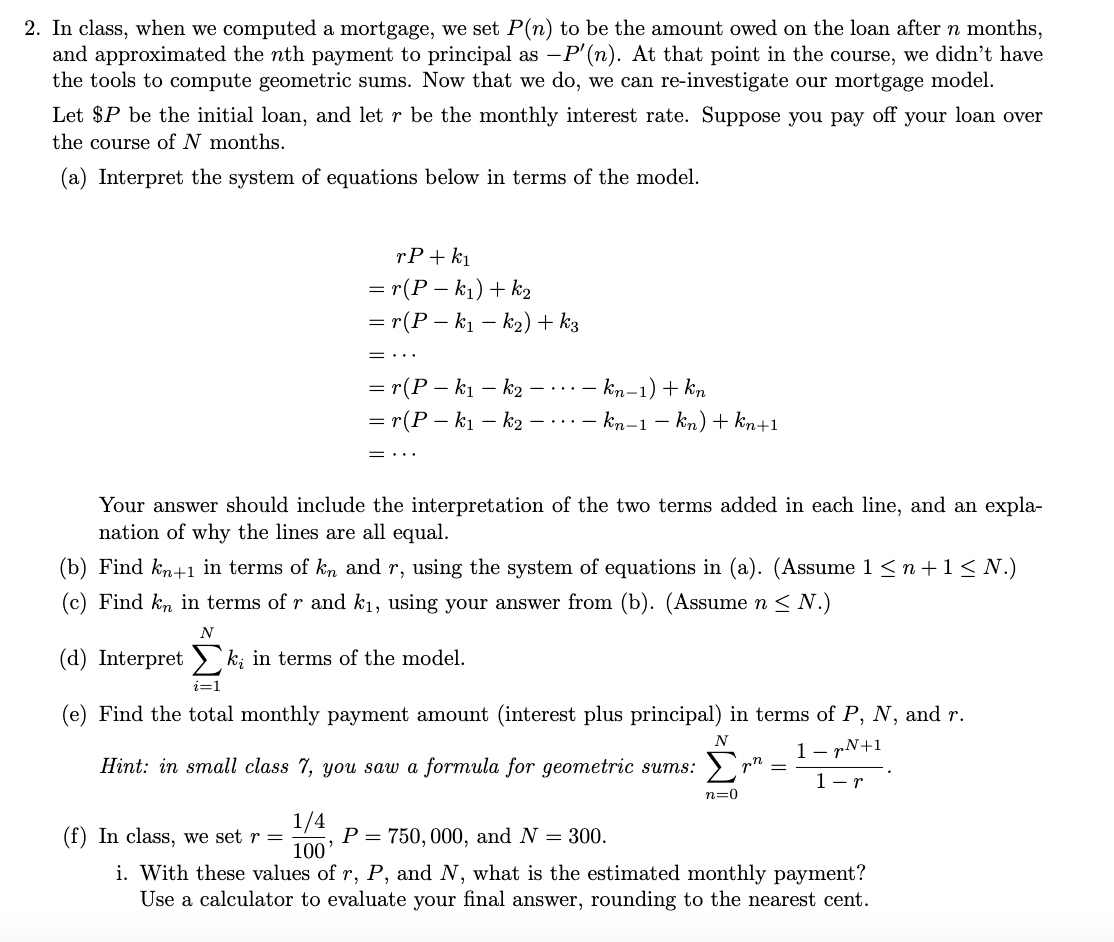# Question 2. In class, when we computed a mortgage, we set $$P(n)$$ to be the amount owed on the loan after $$n$$ months, and approximated the $$n$$th payment to principal as $$-P^{\prime}(n)$$. At that point in the course, we didn't have the tools to compute geometric sums. Now that we do, we can re-investigate our mortgage model. Let $$\ P$$ be the initial loan, and let $$r$$ be the monthly interest rate. Suppose you pay off your loan over the course of $$N$$ months. (a) Interpret the system of equations below in terms of the model. \begin{aligned} & r P+k_{1} \\ = & r\left(P-k_{1}\right)+k_{2} \\ = & r\left(P-k_{1}-k_{2}\right)+k_{3} \\ = & \cdots \\ = & r\left(P-k_{1}-k_{2}-\cdots-k_{n-1}\right)+k_{n} \\ = & r\left(P-k_{1}-k_{2}-\cdots-k_{n-1}-k_{n}\right)+k_{n+1} \\ = & \cdots \end{aligned} Your answer should include the interpretation of the two terms added in each line, and an explanation of why the lines are all equal. (b) Find $$k_{n+1}$$ in terms of $$k_{n}$$ and $$r$$, using the system of equations in (a). (Assume $$1 \leq n+1 \leq N$$.) (c) Find $$k_{n}$$ in terms of $$r$$ and $$k_{1}$$, using your answer from (b). (Assume $$n \leq N$$.) (d) Interpret $$\sum_{i=1}^{N} k_{i}$$ in terms of the model. (e) Find the total monthly payment amount (interest plus principal) in terms of $$P, N$$, and $$r$$. Hint: in small class 7, you saw a formula for geometric sums: $$\sum_{n=0}^{N} r^{n}=\frac{1-r^{N+1}}{1-r}$$. (f) In class, we set $$r=\frac{1 / 4}{100}, P=750,000$$, and $$N=300$$. i. With these values of $$r, P$$, and $$N$$, what is the estimated monthly payment? Use a calculator to evaluate your final answer, rounding to the nearest cent.Transcribed Image Text: 2. In class, when we computed a mortgage, we set $$P(n)$$ to be the amount owed on the loan after $$n$$ months, and approximated the $$n$$th payment to principal as $$-P^{\prime}(n)$$. At that point in the course, we didn't have the tools to compute geometric sums. Now that we do, we can re-investigate our mortgage model. Let $$\ P$$ be the initial loan, and let $$r$$ be the monthly interest rate. Suppose you pay off your loan over the course of $$N$$ months. (a) Interpret the system of equations below in terms of the model. \begin{aligned} & r P+k_{1} \\ = & r\left(P-k_{1}\right)+k_{2} \\ = & r\left(P-k_{1}-k_{2}\right)+k_{3} \\ = & \cdots \\ = & r\left(P-k_{1}-k_{2}-\cdots-k_{n-1}\right)+k_{n} \\ = & r\left(P-k_{1}-k_{2}-\cdots-k_{n-1}-k_{n}\right)+k_{n+1} \\ = & \cdots \end{aligned} Your answer should include the interpretation of the two terms added in each line, and an explanation of why the lines are all equal. (b) Find $$k_{n+1}$$ in terms of $$k_{n}$$ and $$r$$, using the system of equations in (a). (Assume $$1 \leq n+1 \leq N$$.) (c) Find $$k_{n}$$ in terms of $$r$$ and $$k_{1}$$, using your answer from (b). (Assume $$n \leq N$$.) (d) Interpret $$\sum_{i=1}^{N} k_{i}$$ in terms of the model. (e) Find the total monthly payment amount (interest plus principal) in terms of $$P, N$$, and $$r$$. Hint: in small class 7, you saw a formula for geometric sums: $$\sum_{n=0}^{N} r^{n}=\frac{1-r^{N+1}}{1-r}$$. (f) In class, we set $$r=\frac{1 / 4}{100}, P=750,000$$, and $$N=300$$. i. With these values of $$r, P$$, and $$N$$, what is the estimated monthly payment? Use a calculator to evaluate your final answer, rounding to the nearest cent.
Transcribed Image Text: 2. In class, when we computed a mortgage, we set $$P(n)$$ to be the amount owed on the loan after $$n$$ months, and approximated the $$n$$th payment to principal as $$-P^{\prime}(n)$$. At that point in the course, we didn't have the tools to compute geometric sums. Now that we do, we can re-investigate our mortgage model. Let $$\ P$$ be the initial loan, and let $$r$$ be the monthly interest rate. Suppose you pay off your loan over the course of $$N$$ months. (a) Interpret the system of equations below in terms of the model. \begin{aligned} & r P+k_{1} \\ = & r\left(P-k_{1}\right)+k_{2} \\ = & r\left(P-k_{1}-k_{2}\right)+k_{3} \\ = & \cdots \\ = & r\left(P-k_{1}-k_{2}-\cdots-k_{n-1}\right)+k_{n} \\ = & r\left(P-k_{1}-k_{2}-\cdots-k_{n-1}-k_{n}\right)+k_{n+1} \\ = & \cdots \end{aligned} Your answer should include the interpretation of the two terms added in each line, and an explanation of why the lines are all equal. (b) Find $$k_{n+1}$$ in terms of $$k_{n}$$ and $$r$$, using the system of equations in (a). (Assume $$1 \leq n+1 \leq N$$.) (c) Find $$k_{n}$$ in terms of $$r$$ and $$k_{1}$$, using your answer from (b). (Assume $$n \leq N$$.) (d) Interpret $$\sum_{i=1}^{N} k_{i}$$ in terms of the model. (e) Find the total monthly payment amount (interest plus principal) in terms of $$P, N$$, and $$r$$. Hint: in small class 7, you saw a formula for geometric sums: $$\sum_{n=0}^{N} r^{n}=\frac{1-r^{N+1}}{1-r}$$. (f) In class, we set $$r=\frac{1 / 4}{100}, P=750,000$$, and $$N=300$$. i. With these values of $$r, P$$, and $$N$$, what is the estimated monthly payment? Use a calculator to evaluate your final answer, rounding to the nearest cent.&#12304;General guidance&#12305;The answer provided below has been developed in a clear step by step manner.Step1/2(a) The system of equations represents the computation of the remaining amount owed on the loan after each payment is made. The term $rP$ represents the interest accrued on the remaining balance, and $k_n$ represents the amount paid towards the principal in the $n$-th payment. The lines are all equal because each subsequent payment is computed by subtracting the previous payment's principal payment from the remaining balance and then adding the interest accrued on that new balance.(b) Using the system of equations, we can solve for $k_{n+1}$:Explanation:Please refer to solution in this step.Step2/2r(P&#8722;k1&#8203;&#8722;k2&#8203;&#8722;&#8943;&#8722;kn&#8203;)+kn+1&#8203;=r(P&#8722;k1&#8203;&#8722;k2&#8203;&#8722;&#8943;&#8722;kn&#8722;1&#8203;)+kn&#8203;&#8658;kn+1&#8203;=r(P&#8722;k1&#8203;&#8722;k2&#8203;&#8722;&#8943;&#8722;kn&#8203;)+kn&#8203;&#8722;r(P&#8722;k1&#8203;&#8722;k2&#8203;&#8722;&#8943;&#8722;kn&#8722;1&#8203;)(c) We can use the ... See the full answer